Study Guide

# Reciprocal Trigonometric Functions

## Reciprocal Trigonometric Functions

We've covered sine, cosine, and tangent. We're experts on one little piece of trigonometric real estate. (Marvin Gardens? Park Place? Boardwalk? Pull out your Monopoly money.) Kudos to you, but there's more.

Sine, cosine, and tangent each have a reciprocal function. Reciprocal, reciprocity—think of flipping things over, like hamburgers on a grill, pancakes on a griddle, eggs over easy. (When do we eat?) We already know that regular numbers have reciprocals (2 and 1/2 are reciprocals, for example), but we can also flip our trig functions on their heads.

Cosecant is the reciprocal of sine. Its abbreviation is csc. To determine csc, just flip sin over.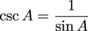Secant is the reciprocal of cosine. Its abbreviation is sec. To determine sec, just flip cos over.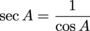Cotangent is the reciprocal of tangent. Its abbreviation is cot. To determine cot, just flip tan over. (Try remembering it all by thinking, "After an evening of sin, Joe stretches out on a cot in the backyard and later flips over to get a better tan." Weird, but it works.)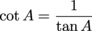And no, they don't sizzle when they're flipped.

### Sample Problem

If a = 8 and b = 15, find the six trig ratios of angle A.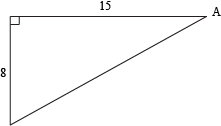We're gonna need that missing hypotenuse, so first use a2 + b2 = c2 to find c.

82 + 152 = c2

64 + 225 = c2

289 = c2

c = 17

Now let's plug those sides into our relationships for sin, cos, and tan. Remember SOHCAHTOA. We're looking at angle A, so the opposite side is 8, the adjacent side is 15, and the hypotenuse is 17. Ready, go.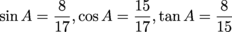Now we take the reciprocals, or flip each function over.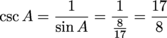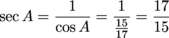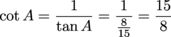### Sample Problem

What are the six trig ratios of angle B?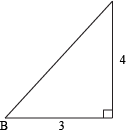Once again, we'll need to track down that hypotenuse before we can take a trip to TrigVille. Give your buddy Pythagoras a call.

a2 + b2 = c2

We know the two legs of the triangle, so plug 'em in for a and b.

32 + 42 = c2

9 + 16 = c2

25 = c2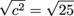c = 5

Next, find the sine, cosine, and tangent of angle B.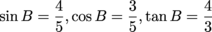To find the reciprocals, just flip the fractions over.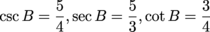### Sample Problem

An isosceles right triangle has two legs with a length of 1. If angle A is one of the non-right angles, what are the sine, cosine, tangent, cosecant, secant, and cotangent of angle A?

"Isosceles" looks pretty weird, but it really just means both legs have the exact same length. We don't have a picture to help us out, but who needs pictures? We're math detectives up in here.

All right, so we know both legs, which means a = 1 and b = 1 in our Pythagorean Theorem. Let's find c.

12 + 12 = c2

1 + 1 = c2

c2 = 2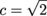There's our hypotenuse. Now, we know angle A is not the triangle's right angle. It's one of the other guys, which means its opposite side and adjacent side are both 1. Apply the trig ratios, and don't forget to rationalize your denominators.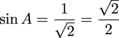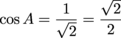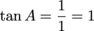When flipping over your fractions to find the reciprocals, use the original fraction (with the pre-rationalized denominator). It will save you time and heartache.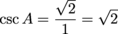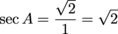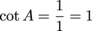• ### Co-functions

No, you won't stumble over co-functions if you find yourself in Dysfunction Junction. They're deeply embedded in trigonometric functions. Did you notice the "co" in cosine, cosecant, and cotangent?

"Co" is short for complementary.

Co + function = complementary functions.

Does the word complementary ring a bell? In geometry, we learned that complementary angles combine to form a 90° angle.

We also know that the sum of the angles in a triangle must be 180°. So if angle C is a right angle (90°), the other two angles must add up to the other 90°.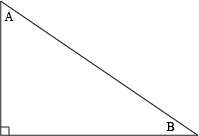Since A + B = 90°, A and B are complementary angles.

And by rearranging things a little, we can see that:

B = 90° – A

and

A = 90° – B

Now let's apply our "co" to the trig functions.

Cosine is the complementary function of sine.

Cosecant is the complementary function of secant.

Cotangent is the complementary function of tangent.

What's this all about? Here's the deal. Take another peek at our triangle:In this triangle, the cosine of angle A is the same thing as the sine of angle B.

cos A = sin B

Go ahead and let that sink in for a sec. Cosine is the adjacent side over the hypotenuse (CAH), and sine is the opposite side over the hypotenuse (SOH). But the side adjacent to angle A is the same thing as the side opposite angle B. That's why cos A = sin B. They give us the exact same ratio.

Here's where the co-function stuff comes in. Substituting in B = 90° – A gives us:

cos A = sin(90° – A)

See what we did there? The cosine of A is the same as the sine of A's complementary angle.

Similarly:

csc A = sec B
csc A = sec(90° – A)

And:

cot A = tan B
cot A = tan(90° – A)

It works the other way, too:

sin A = cos B = cos(90° – A)
sec A = csc B = csc(90° – A)
tan A = cot B = cot(90° – A)

### Sample Problem

Write sin(30°) in terms of its co-function.

This is way more chill than it might sound. The sine's co-function is just the cosine. Using sin A = cos(90° – A), we get:

sin(30°) = cos(90° – 30°)

sin(30°) = cos(60°)

Yep, that's it. The sine of 30° is the exact same thing as the cosine of 60°. Plop 'em into a calculator if you don't believe us.

### Sample Problem

Write csc(70°) in terms of its co-function.

Cosecant goes hand-in-hand with secant, so we'll use complementary angles again. The formula this time is csc A = sec(90° – A).

csc(70°) = sec(90° – 70°)
csc(70°) = sec(20°)

### Sample Problem

Write tan(10°) in terms of its co-function.

We wanna turn that tangent into cotangent, so we'll use the formula tan A = cot(90°– A).

tan(10°) = cot(90° – 10°)
tan(10°) = cot(80°)

### Special Triangles

In trig and—eventually—calculus, you'll find that we use certain angles over and over, ad infinitum, ad nauseam. These angles make up two special triangles. We'll take a look at special triangles in the next section.

### 45°-45°-90° Triangles

If we're handling a 45° angle in trig, we need to be able to draw this reference triangle.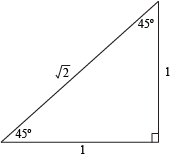The trick? First, draw an isosceles right triangle. Isosceles triangles have two legs that are the same length.

Label each leg 1. Like this: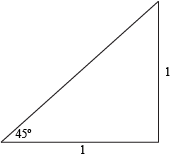Then, thanks to the Pythagorean Theorem, we can find the hypotenuse:

12 + 12 = c2
1 + 1 = c2
c2 = 2And since we've got one 45° angle and one 90° angle, we know the third angle must be 180° – 90° – 45° = 45°.We clearly know a heck of a lot more than when we started. Not only do we know about trig functions, but we also know about their complementary functions. Go us. Now, here's how we apply it in a triangulated world.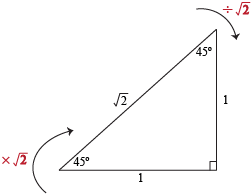When going from the hypotenuse to a leg → divide by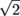.

When going from a leg to the hypotenuse → multiply by.

These tricks work for any 45°-45°-90° triangle, no matter how long its sides are. A triangle with two 45° angles and one 90° is always gonna be isosceles, which means it'll always have two equal legs.

### Sample Problem

Find x.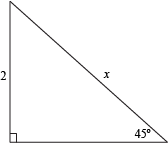First, rotate the reference triangle to match the problem triangle.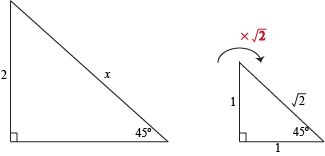We have the leg. Now we need the hypotenuse. Multiply by. The answer is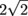. Note that we also could've used the Pythagorean Theorem if we wanted, but this way was quicker.

### Sample Problem

Find x.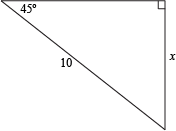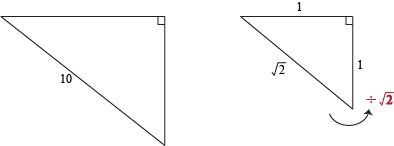We have the hypotenuse. Now we need a leg. Divide by.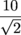Now we just need to rationalize the denominator. You know how math teachers hate having a radical sign down in the basement.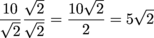### Sample Problem

Find x.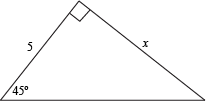This one is a cinch. Since we've got a 45° angle and a 90° angle, that must mean we have an isosceles triangle (equal-length legs, remember?). The answer is 5.

### 30°-60°-90° Triangles

If we're working with a 30° or 60° angle, we need to be able to draw this reference triangle.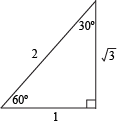To sketch this bad boy, first draw an equilateral triangle and label each side with a 2. Like this: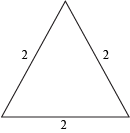Now, split the triangle in half by bisecting the vertex angle. Label each half of the base with a 1. Like this: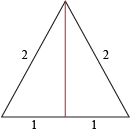Now, find the height, h. That's the red line. It's also the leg of a right triangle, so we can use our main dude Pythagoras.

12 + h2 = 22
h2 = 22 – 12
h2 = 4 – 1
h2 = 3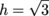Victory. You've got your 30°-60°-90° reference triangle.

Here's how all this applies in the trig world.The short leg is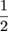the length of the hypotenuse. Or, if you're an optimist, the hypotenuse is twice the length of the short leg. BTW, the short leg is always the side opposite the 30° angle.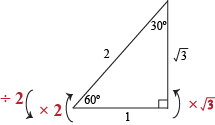To go from the short leg to the hypotenuse → multiply by 2.

To go from the hypotenuse to the short leg → divide by 2.

To go from the short leg to the long leg → multiply by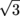.

To go from the long leg to the short leg → divide by.

If we have the long leg and need the hypotenuse, we'll need to go the long way around. Find the short leg first, and then find the hypotenuse.

If you have the hypotenuse and need the long leg, find the short leg first, and then find the long leg.

Man, this is sounding like a LEGO marathon or a daddy long legs convention—with numbers. Hang in there.

### Sample Problem

Find x.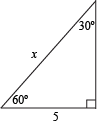First, rotate the reference triangle and sketch it nearby. Like this.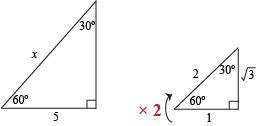We have the short leg and need the hypotenuse. Multiply the short leg by 2. The answer is 10.

### Sample Problem

Find x.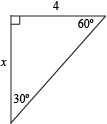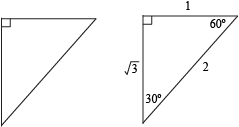We have the short leg and need the long leg. Multiply by. The answer is 4. We could do this all day.

### Sample Problem

Find x.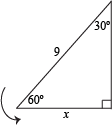We have the hypotenuse and need the short leg, so we've gotta divide by 2.

x = 92 = 4.5